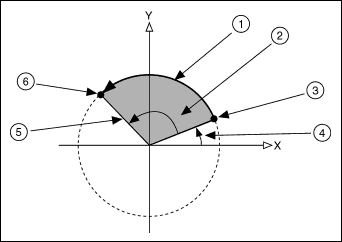# Circular Interpolation

Publish Date: Sep 02, 2016 | 10 Ratings | 2.70 out of 5 | Print

The FlexMotion controller family supports hardware-based circular interpolation. Since a circle is a two-dimensional object, circular motion requires two motors moving synchronously. Specifically, the motors need to have sinusoidal profiles that are 90 degrees out of phase with each other. For each motor to follow a sinusoidal position profile, each point along its path must be calculated based on the parameters for the circle: the circle's radius, its start angle, and its travel angle.

Radius is the arc radius in counts (servo axes) or steps (stepper axes). The range is 2 to (2^31) ±1 counts (steps).

Start angle is the double precision floating point value in degrees of the starting angle of the arc. The range is 0 to 360° where angle 0 is along the positive X axis and values increase counter-clockwise from the positive X axis in the X-Y plane.

Travel angle is the double precision floating point value in degrees of the angle to be traversed. The range is -1,474,560 to +1,474,200° (-4,096 to +4,095 revolutions). A positive Travel Angle defines counter-clockwise rotation in the X-Y plane.

Components of a circular move.1. Circular arc
2. Travel angle
3. Starting position
4. Start angle
5. Radius
6. Ending position

In order to perform these calculations fast enough to provide smooth motion and zero chordal error, the calculations are performed by the FlexMotion controller itself rather than by the host computer. The trajectory generator on the FlexMotion board calculates each of those points in real-time and splines between them to form a smooth circular path. This frees the processor on the computer from having to do all of the intensive calculations necessary to create a circle.

See the descriptions for the Load Circular Arc, Load Spherical Arc, and Load Helical Arc in the FlexMotion software and VI reference information for more information on this important feature of the FlexMotion controllers.

### Ratings

Rate this document

Answered Your Question?
Yes No